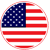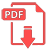# Home | Mathematics | Geometry(Stereometry)

In the previous two sections we’ve looked at lines and planes in three dimensions (or R3) and while these are used quite heavily at times in a Calculus class there are many other surfaces that are also used fairly regularly and so we need to take a look at those.

In this section we are going to be looking at quadric surfaces. Quadric surfaces are the graphs of any equation that can be put into the general form
Ax2+By2+Cz2+Dxy+Exz+Fyz+Gx+Hy+Iz+J=0

where A, … , J are constants.

There is no way that we can possibly list all of them, but there are some standard equations so here is a list of some of the more common quadric surfaces.EnglishArmenianFrench